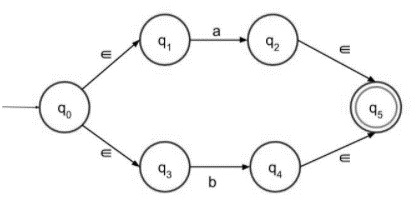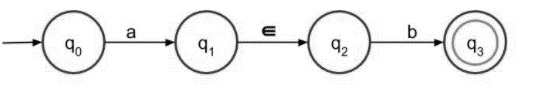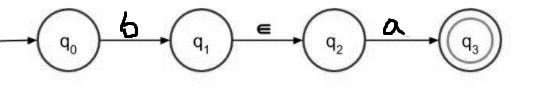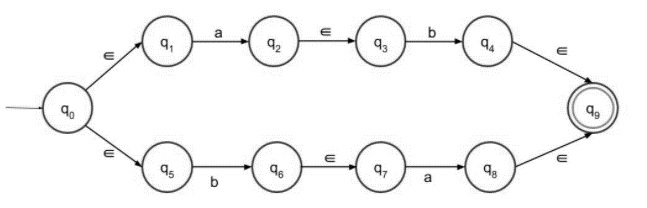# Construct âˆˆ-NFA of Regular Language L = {ab,ba}

The ε transitions in Non-deterministic finite automata (NFA) are used to move from one state to another without having any symbol from input set Σ

ε-NFA is defined in five tuple representation

{Q, q0, Σ, δ, F}

Where,

• δ − Q × (Σ∪ε)→2Q

• Q − Finite set of states

• Σ − Finite set of the input symbol

• q0 − Initial state

• F − Final state

• δ − Transition function

NFA and NFA with epsilon both are almost the same; the only difference is their transition function.

NFA transition function is as follows −

δ − Q X Σ→ 2Q

NFA with ε- transition functions is as follows −

δ: Q × (Σ∪ε)→2Q

Construct NFA with epsilon for a given language L= {ab, ba}.

Follow the steps given below −

Step 1 − NFA with epsilon for (a+b) is given below −It accepts either a or b as an input, and both go to the final state.

Step 2 − NFA with epsilon for ab is as follows −Concatenating a and b with epsilon, and a must followed by b then only it can reach the final state.

Step 3 − NFA with epsilon for ba is as follows −Concatenating b and a with epsilon, and b must follow by a then only it can reach the final state.

Step 4 − Now the final NFA with epsilon for the language L={ab, ba}.

The language consists of strings ab or ba, it can be written as (ab + ba). So the final ε-NFA having two paths, one path is for ab and another path is for ba both goes to the final state.

In the above steps we individually construct the structures for ab and ba. Now, add those two structures to get our result.